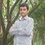# Help to Evaluate an Integral

I Need Help in evaluating this integral

$\int\limits_0^\frac{\pi}{2} \frac {\theta cos \theta d\theta } { sin \theta + \sin ^ 3 \theta }$

None of my teachers were able to solve this, nor my friends. It has a finite answer, as one of my friends checked on a scientific calculator.Note by Ghanashyam Chakravarthi
6 years, 9 months ago

This discussion board is a place to discuss our Daily Challenges and the math and science related to those challenges. Explanations are more than just a solution — they should explain the steps and thinking strategies that you used to obtain the solution. Comments should further the discussion of math and science.

When posting on Brilliant:

• Use the emojis to react to an explanation, whether you're congratulating a job well done , or just really confused .
• Ask specific questions about the challenge or the steps in somebody's explanation. Well-posed questions can add a lot to the discussion, but posting "I don't understand!" doesn't help anyone.
• Try to contribute something new to the discussion, whether it is an extension, generalization or other idea related to the challenge.

MarkdownAppears as
*italics* or _italics_ italics
**bold** or __bold__ bold
- bulleted- list
• bulleted
• list
1. numbered2. list
1. numbered
2. list
Note: you must add a full line of space before and after lists for them to show up correctly
paragraph 1paragraph 2

paragraph 1

paragraph 2

[example link](https://brilliant.org)example link
> This is a quote
This is a quote
    # I indented these lines
# 4 spaces, and now they show
# up as a code block.

print "hello world"
# I indented these lines
# 4 spaces, and now they show
# up as a code block.

print "hello world"
MathAppears as
Remember to wrap math in $$ ... $$ or $ ... $ to ensure proper formatting.
2 \times 3 $2 \times 3$
2^{34} $2^{34}$
a_{i-1} $a_{i-1}$
\frac{2}{3} $\frac{2}{3}$
\sqrt{2} $\sqrt{2}$
\sum_{i=1}^3 $\sum_{i=1}^3$
\sin \theta $\sin \theta$
\boxed{123} $\boxed{123}$

Sort by:

First, use IBP and we'll end up with $\int_{0}^{\frac{\pi}{2}}\dfrac{x \cos{x}}{\sin{x}+\sin^{3}{x}}\, dx = -\frac{\pi}{4}\log{2} - \int_{0}^{\frac{\pi}{2}} \left( \log{\sin{x}} - \frac{\log{(1+\sin^{2}{x})}}{2}\, dx \right)$

and since $\int_{0}^{\frac{\pi}{2}} \log{\sin{x}}\, dx = -\frac{\pi}{2}\,\log{2}$

we have $\int_{0}^{\frac{\pi}{2}}\dfrac{x \cos{x}}{\sin{x}+\sin^{3}{x}} \, dx = \frac{\pi}{4}\,\log{2} + \int_{0}^{\frac{\pi}{2}} \frac{\log{(1+\sin^{2}{x})}}{2}\, dx \quad\cdots\cdots\cdots\cdots (0)$

Let $I(k) = \int_{0}^{\frac{\pi}{2}} \frac{\log{(1+\sin^{2}{x}+k\, \sin^{2}{x})}}{2}\, dx \quad\cdots\cdots\cdots\cdots (1)$

To evaluate I(k), we can make use of differentiation under the integral sign:

$\frac{\partial I}{\partial k} = \int_{0}^{\frac{\pi}{2}} \frac{\sin^{2}{x}\, dx}{1+\sin^{2}{x}+k\, \sin^{2}{x}}$ $\implies I'(k) = \int_{0}^{\frac{\pi}{2}} \frac{1}{k+1}\left(1-\frac{\sec^{2}{x}}{1+(k+2)\tan^{2}x}\right)\, \, dx$ $=\frac{x}{k+1}-\frac{\tan^{-1}{(\sqrt{k+2}\,\tan{x})}}{(k+1)\, \sqrt{k+2}}\bigg|_0^{\frac{\pi}{2}} = \frac{\pi}{2\,k+2}\left(1-\frac{1}{\sqrt{k+2}}\right)$

Integrating w.r.t. k, we get:

$I(k)= \frac{\pi}{2}\left(\log{(k+1)}-\log{\left(\frac{\sqrt{k+2}-1}{\sqrt{k+2}+1}\right)}\right)+C=\frac{\pi}{2}\, \log{\left(2\, (\sqrt{k+2}+1)^{2}\right)}+C$ $\therefore I(k) = \frac{\pi}{2}\, \log{\left(2\, (\sqrt{k+2}+1)^{2}\right)}+C \quad\cdots\cdots\cdots\cdots (2)$

To find C, substitute k=-1. From (1), $I(-1) = 0$ and from (2),
$I(-1) = \frac{3\,\pi}{2}\log{2}+C$ $\implies C= - \frac{3\,\pi}{2}\log{2}$ The required integral is $I(0) = \int_{0}^{\frac{\pi}{2}} \log{(1+\sin^{2}{x})}\, dx = \frac{\pi}{2}\, \log{\left(2\, (\sqrt{2}+1)^{2}\right)} - \frac{3\,\pi}{2}\log{2}$

Substituting in (0), we see that $\int_{0}^{\frac{\pi}{2}}\dfrac{x \cos{x}\, dx}{\sin{x}+\sin^{3}{x}} = \frac{\pi}{4}\,\log{2} + \frac{\pi}{4}\, \log{\left(2\, (\sqrt{2}+1)^{2}\right)}- \frac{3\,\pi}{4}\log{2}$ $= \boxed{{\frac{\pi}{4}\log{\left(\frac{3+2\,\sqrt{2}}{2}\right)}}}$

- 6 years, 9 months ago

its an awesome solution ..!!

- 6 years, 9 months ago

Hello... Where is d(theta)? This is normally written when integrating functions to say reverse the process and of course, finding the antiderivative. Also is theta really before cos(theta)?

- 6 years, 9 months ago

Thanks John. I had forgotten d(theta) in the process of tying in Latex. And yes there is a (theta) before cos(theta). I've made the change.

- 6 years, 9 months ago

I tried using limits. What's the answer? Is it by any chance 0??

- 6 years, 9 months ago

I don't know the answer, but I don't think its zero.

- 6 years, 9 months ago

Look,at first the sum looks easy,doesn't it? my answer was (pi/2) ln(tan(pi/2)/tan 0)) Now as this tan((pi/2)/tan(0)) is undefined. so lets find limit x->0 tan((pi/2)+x)/tan(x). As this is (infinity)/(infinity) form, we can differentiate on numerator and denominator according to L'Hospital Rule. Doing so, and putting x=0,once the expression is defined, we get the limiting value to be 1. So putting this limiting value in the initial expression, answer is (pi/2)(ln 1) or, (pi/2). 0 or 0. In the end answer is coming out to be 0. Did I go wrong anywhere?

- 6 years, 9 months ago

How did you get (pi/2) ln(tan(pi/2)/tan 0)) ? From there on its correct.

- 6 years, 9 months ago

That is very simple. from the denominator take sine theta common. put cos^2(theta)
in place of 1+ sin^2(theta). Then do the required cancellation to get expression as ((theta)/(sin (theta).cos(theta))) inside the integral. Now apply,the rule for definite integral: Integral f(x)from 0 to a=integral f(a-x)from 0 to a. Put the limits from 0 to pi/2.Then you will get the final expression : pi/2(sec^2(theta)d(theta)/tan(theta)) Now you should be able to do the rest. Its simple enough .

- 6 years, 9 months ago

but 1- sin^2 (theta) is cos^2(theta) !!!

- 6 years, 9 months ago

you can solve this by using Wallis fomula

- 6 years, 9 months ago

hey can you tell me how..

- 6 years, 9 months ago